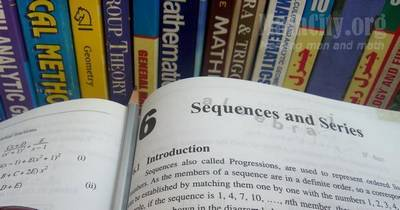# Chapter 06: Sequences and SeriesNotes (Solutions) of Chapter 06: Sequences and Series, Text Book of Algebra and Trigonometry Class XI (Mathematics FSc Part 1 or HSSC-I), Punjab Text Book Board, Lahore.

• Introduction
• Types of Sequences
• Exercise 6.1
• Arithmetic Progression(A.P)
• Exercise 6.2
• Aritmetic Mean (A.M)
• n Arithmetic Means between two given Numbers
• Exercise 6.3
• Series
• Exercise 6.4
• World Problems on A.P.
• Exercise 6.5
• Geometric Progression(G.P)
• Exercise 6.6
• Geometric Means
• n Geometric Means between two given numbers
• Exercise 6.7
• Sum of n terms of a Geometric Series
• The Infinite Geometric Series
• Exercise 6.8
• World Problems on G.P.
• Exercise 6.9
• Harmonic Progression(H.P)
• Harmonic Means
• n Harmonic Means between two numbers
• Relation between Arithmic, Geometric and Harmonic Means
• Exercise 6.10
• Sigma Notation(or Summation Notation)
• To find the Formulae of Sum
• Exercise 6.11

Exercise 6.2⇒ Question 11(i)

If $l,m,n$ are the $p$th, $q$th, $r$th terms of an A.P., show that $$l(q-r)+m(r-p)+n(p-q)=0$$ Solution: Let $a_1$ be first term and $d$ be common difference of A.P, then \begin{align}l=a_1+(p-1)d,\\ m=a_1+(q-1)d,\\ n=a_1+(r-1)d.\end{align} Now \begin{align}L.H.S &= l(q-r)+m(r-p)+n(p-q)\\ &= lq-lr+mr-mp+np-nq\\ &=(l-n)q+(m-l)r+(n-m)p\\ &=(a_1+(p-1)d-a_1-(r-1)d)q+( a_1+(q-1)d-a_1-(p-1)d)r\\ &\,\,+(a_1+(r-1)d-a_1-(q-1)d)p\\ &=((p-1)d-(r-1)d)q+( (q-1)d-(p-1)d)r+((r-1)d-(q-1)d)p\\ &=(p-1-r+1)dq+(q-1-p+1)dr+(r-1-q+1)dp\\ &=(p-r)dq+(q-p)dr+(r-q)dp\\ &=[pq-qr+qr-pr+pr-pq]d=(0)d=0=R.H.S\end{align}

Exercise 6.4⇒ Question 3(ii)

An equation $3n^2-17n-288=0$ should be $3n^2-17n-228=0$. … suggested by Abdullah Zafar (Garrison Academy Kharian)

• Exercise 6.3 | View Online | Download PDF (123KB)The following short questions was send by Mr. Akhtar Abbas.

• fsc/fsc_part_1_solutions/ch06